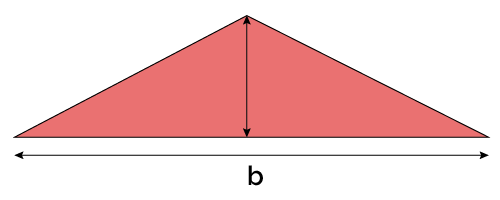# Area of an Obtuse Triangle Calculator

Created by Aleksandra Zając, MD
Reviewed by Rijk de Wet
Last updated: May 31, 2022

If the basic triangle area formula is not enough, the area of an obtuse triangle calculator is made for you. Reading on, you'll find out:

• How to calculate the area of an obtuse triangle;
• What are the area of obtuse triangle formulas — for every occasion;
• How many types of obtuse triangles are there.

## How to define an obtuse triangle? The characteristics of an obtuse triangle

An obtuse triangle is a triangle with one interior angle greater than 90° (degrees).

The characteristics of an obtuse triangle are:

• One interior angle is greater than 90° (90 degrees being a right angle);
• The rest of the angles are under 90° (acute angles);
• The side opposite the obtuse angle is the longest side of the triangle;
• Can be a scalene or an isosceles triangle, but can never be an equilateral triangle.

## Using the area of an obtuse triangle calculator

To use our obtuse triangle calculator:

2. Choose the mode that you want to use — it depends on what you already know.

• Let's assume we know the length of two sides and an angle between them. We have to choose the SAS mode.
3. Input the length of the sides and a measure of the angle between them. You can choose the units for the most convenient for you and switch them at any time.

4. The result appears immediately in the last row of the calculator.

5. If you want to change the mode, choose another one from the drop-down list — no need to restart the calculator.

6. Now you know how to use the area of an obtuse triangle calculator!

## Area of an obtuse triangle — formulas

There are four ways to find the area of an obtuse triangle. Which you use depends on which data you have given. You can calculate the area of an obtuse triangle with sides and angles, with sides only, or with the height and base.

The area of obtuse triangle formulas based on what data is known are as follows:

1. Base ($b$) and height ($h$)
$\footnotesize\quad \text{area}=\frac{1}{2} \times b \times h$
1. Three sides ($a$, $b$, $c$)
\footnotesize\quad \begin{align*} \text{area} &= \frac{1}{4} \times \sqrt{(a+b+c)}\ \times\\[1em] &\quad\sqrt{(-a+b+c)(a-b+c)}\ \times\\[1em] &\quad\sqrt{(a+b-c)} \end{align*}
1. Two sides and the angle between them (sides $a$ and $b$ and angle $\gamma$)
$\footnotesize\quad \text{area}=\frac{1}{2} \times a \times b \times \sin(\gamma)$
1. Two angles ($\gamma$ and $\beta$) and a side between them ($a$)
$\footnotesize\quad \text{area} = a^2\!\times\! \sin(\beta)\! \times\! \frac{\sin(\gamma)}{2\! \times \sin(\beta + \gamma)}$

## More triangle area calculators

Check out the rest of our triangle area calculators collection:

## FAQ

### How do I count the area of an obtuse triangle with sides 10, 17, and 25 inches?

The area of this triangle is 61.19 in². To find the triangle area with three sides:

1. Use Heron's formula:
area = 0.25 × √[(a + b + c)(−a + b + c)(a − b + c)(a + b − c)]
2. Knowing all three a, b, and c, we can solve the equation, and realize that...
3. The answer is 61.19 in².

### How do I calculate the area of an obtuse triangle with sides and angles?

To count the area with two sides and an angle between them, use the formula:

• area = 0.5 × a × b × sin(γ), where a, b are the sides and γ is the angle between those sides.

To calculate the area with two angles and a side between them, use the equation:

• area = a² × sin(β) × sin(γ) / [2 × sin(β + γ)], where β and γ are the angles, and a is the side between those angles.

### How many types of obtuse triangles are there?

There are two main types of obtuse triangles:

• Isosceles — with two sides equal;
• Scalene — with no sides equal.

### How do I calculate the area of an obtuse triangle with two angles 97° and 34°, and the side between them 13 inches?

The area of this triangle is 62.14 in². To calculate it step by step:

1. Use the formula area = a² × sin(β) × sin(γ) / [2 × sin(β+γ)], where β and γ are the angles, and a is the side between those angles.
2. Solve the equation: area = 13² × sin(97) × sin(34) / [2 × sin(97+34)].
3. The area of this triangle is 62.14 in².
Aleksandra Zając, MD
Given
base and heightBase
in
Height
in
Area
in²
People also viewed…

### Circle skirt

Circle skirt calculator makes sewing circle skirts a breeze.

### Cross product

Cross product calculator finds the cross product of two vectors in a three-dimensional space.

### Grams to cups

The grams to cups calculator converts between cups and grams. You can choose between 20 different popular kitchen ingredients or directly type in the product density.

### Lateral surface area of a cylinder

Our lateral surface of a cylinder calculator can help youth calculate a cylinders's lateral surface.